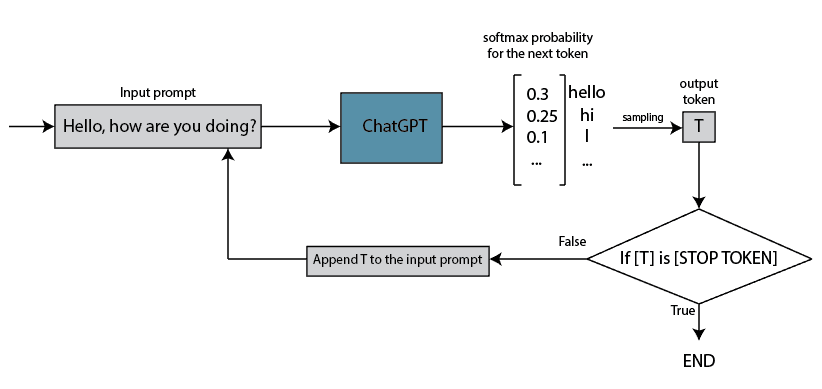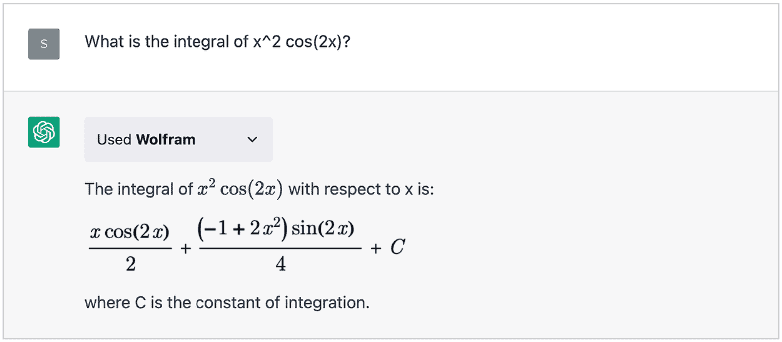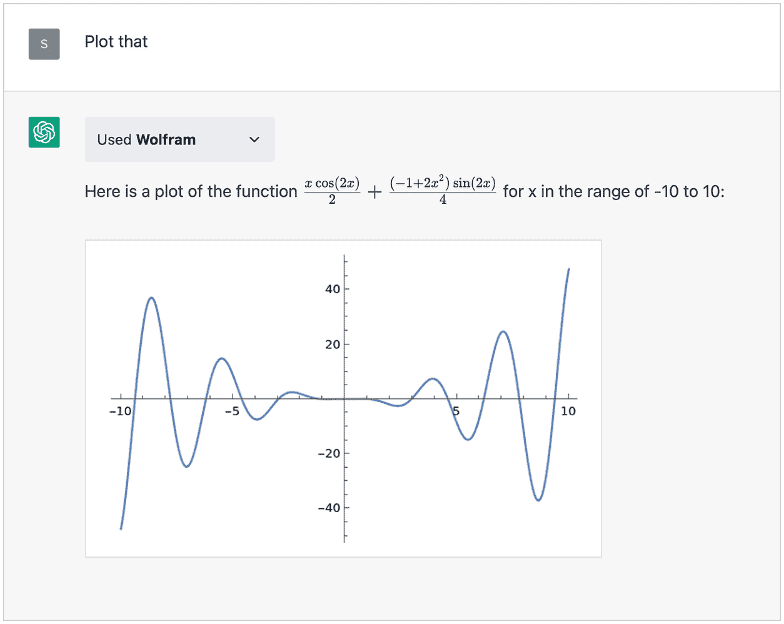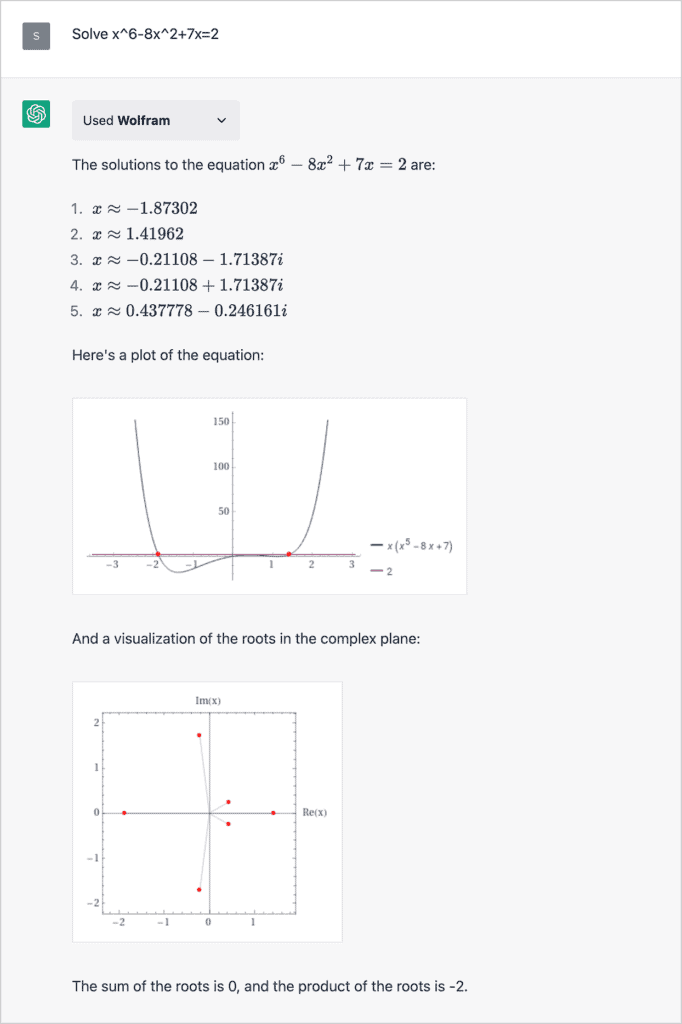## 1. Introduction

In this tutorial, we’ll discuss why ChatGPT is bad at math. While ChatGPT excels at handling a wide range of intricate questions, it’s interesting that it struggles with basic logic problems.

We’ll explore the reasons behind this issue and how an enhanced version of ChatGPT can overcome these challenges.

## 2. What Is CHATGPT?

ChatGPT is a large language model (LLM) developed by OpenAI. It’s based on the GPT (Generative Pre-trained Transformer) architecture. It generates human-like text responses in conversational settings and understands various topics and writing styles.

Using deep learning techniques, ChatGPT learns patterns and structures from the text it’s trained on. That knowledge generates coherent and contextually relevant responses to user inputs. ChatGPT can engage in dialogue, answer questions, provide explanations, generate creative text, and assist with various language-related tasks.

While ChatGPT has impressive capabilities, it’s important to note that it may sometimes produce incorrect or nonsensical responses. It relies on patterns and associations learned from training data and may not always possess accurate or up-to-date information. Also, ChatGPT primarily focuses on language understanding and generation rather than solving math and logic problems.

## 3. Why Is ChatGPT Bad at Math?

There are several reasons why ChatGPT is bad at solving math and logic tasks in general. Here, we’ll discuss some of the most important.

### 3.1. Training Data

First of all, the primary reason for ChatGPT’s difficulty with math is its training data. While it has been exposed to a vast amount of internet text, the training data isn’t specifically geared toward mathematical concepts and problem-solving. As a result, ChatGPT may lack the necessary mathematical knowledge and reasoning abilities required to handle complex math problems.

### 3.2. ChatGPT Architecture

Another crucial factor is the architecture of the GPT model itself. GPT is primarily designed for language understanding and generation.

Its focus is on processing and generating coherent human-like text, which makes it well-suited for tasks like language translation or text generation. However, math involves precise calculations, logic, and formal reasoning, which differ from language tasks. The GPT model’s architecture may not be optimized for these specific mathematical operations.

Moreover, math problems often require a deeper understanding of concepts and a step-by-step reasoning process to arrive at accurate solutions. While ChatGPT may excel at generating plausible responses, it may struggle to produce accurate mathematical results due to a lack of formal understanding and the absence of a mechanism to perform mathematical computations.

### 3.3. ChatGPT Probabilistic Nature

ChatGPT is a probability-based generative model. It generates text responses from a softmax function probability distribution. Moreover, in one iteration through the model, it outputs only a single token, and the sampling of that token is performed from the probability distribution generated by the softmax function:Because of its probabilistic nature, ChatGPT introduces an element of uncertainty in its responses. For math problems, where precision and correctness are crucial, relying solely on a probabilistic language model may not be ideal.

## 4. Can ChatGPT Be Good at Math?

The short answer is “Yes”, it can be, and it’ll be in the future. While the base version of ChatGPT may have limitations in handling complex math problems, it’s possible to fine-tune and customize the model to improve its mathematical capabilities.

### 4.1. How Much Better Is GPT-4 at Math?

For instance, the GPT-4 version released on 14 March 2023 showed significant improvements in solving math problems. This version of ChatGPT is not available as a free service, and we need to pay a monthly subscription to use it. Also, GPT-4 has aroundmore parameters than ChatGPT, and it can accept images together with text as input.

Researchers from OpenAI tested GPT-4 on various professional and academic benchmarks, achieving human-level performance in many of them. The GPT-4 technical report describes the entire process, and we’ll mention only specific math and logic task results in this section.

For example, GPT-4 ranked in the top 11% of scores on the SAT Math Test by solving 700 out of 800 tasks. The SAT Math Test evaluates how well we can utilize mathematical concepts and skills to solve problems commonly encountered in college and professional environments.

Also, it’s interesting that GPT-4 solved onlyout oftasks from AMC(American Mathematics Competition) and solvedout oftasks from AMC. It means that it performed around the median in the AMCand in the bottomin the AMC. The AMCis for students in 10th grade and below and covers the high school curriculum up to 10th grade. The AMCcovers the entire high school curriculum, including trigonometry, advanced algebra, and advanced geometry, but excluding calculus.

Lastly, it’s worth mentioning that researchers tested GPT-4 with Leetcode problems, and it solved/easy,/medium, and/hard problems.

### 4.2. ChatGPT Wolfram Plugin

Plugins are tools designed for ChatGPT which help access up-to-date information, run computations, or use third-party services. Basically, those are ChatGPT extensions that help it solve some particular problems. In our context, we’re interested in the Wolfram plugin. It allows ChatGPT to access computation, math, curated knowledge, and real-time data through Wolfram Alpha and Wolfram Language.

Wolfram Alpha is a computational knowledge engine, specifically popular in the mathematical community and used for solving diverse math problems. Besides mathematics, it covers an extensive array of domains, including physics, chemistry, engineering, finance, geography, linguistics, and many others.

The combination of ChatGPT and Wolfram Alpha brings together the probabilistic language generation of ChatGPT with Wolfram Alpha’s computational knowledge and natural language understanding. This combination looks like an expert who understands math problems, uses Wolfram Alpha to solve them, and presents solutions back with some extended explanation.

For example, it easily solves integrals:And even can plot the result:Also, it can solve some complex equations and many more:## 5. Conclusion

In this article, we’ve explored the limitations of ChatGPT in handling math-related tasks. It’s crucial to note that the free version of ChatGPT has difficulties with math and logic problems.

However, the paid version, GPT-4, along with the integration of the Wolfram plugin, offers the capability to solve a wide range of math problems.